# Chapter 10 Constructions NCERT Exemplar Solutions Exercise 10.4 Class 10 MathsChapter Name NCERT Maths Exemplar Solutions for Chapter 10 Construction Exercise 10.1 Book Name NCERT Exemplar for Class 10 Maths Other Exercises Exercise 10.1Exercise 10.2Exercise 10.3 Related Study NCERT Solutions for Class 10 Maths

### Exercise 10.4 Solutions

1. Two line segments AB and AC include an angle of 60° where AB = 5 cm and AC = 7 cm. Locate points P and Q on AB and AC, respectively such that AP = (3/4)AB and AQ = (1/4)AC. Join P and Q and measure the length PQ.

Solution
Steps of construction:

1. Draw a line segment AB = 5 cm.
2. Also, make ∠BAZ = 60°.
3. With center A and radius 7 cm, draw an arc cutting the line AZ at C.
4. Draw a ray AX, making an acute ∠BAX.
5. Divide AX into four equal parts, namely AA1 = A1A2 = A2A3  = A3A4 .
6. Join A4B.
7. Draw A3P || A4B meeting AB at P.
8. Therefore, P is the point on AB such that AP = (3/4) AB.
9. Now, draw a ray AY, such that it makes an acute ∠CAY.
10. Divide AY into four parts, namely AB1 = B1B2 = B2B3 = B3B4 .
11. Join B4C.
12. Draw B1Q || B4C meeting AC at Q. We get, Q is the point on AC such that AQ = (1/ 4)AC.
13. Join PQ and measure it.
14. PQ = 3.25 cm.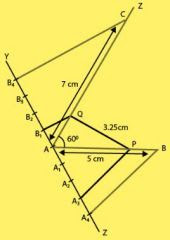2. Draw a parallelogram ABCD in which BC = 5 cm, AB = 3 cm and angle ∠ABC = 60° , divide it into triangles BCD and ABD by the diagonal BD. Construct the triangle BD'C' similar to triangle BDC with scale factor 4/3. Draw the line segment D'A' parallel to DA where A' lies on extended side BA. Is A'BC'D a parallelogram ?

Solution

Steps of constructions:

1. Draw a line AB = 3 cm.
2. Now draw a ray BY making an acute ∠ABY=60°.
3. With centre B and radius 5 cm, draw an arc cutting the point C on BY.
4. Draw a ray AZ making an acute ∠ZAX’=60°
(BY||AZ, as, ∠YBX’=ZAX’=60°)
5. With centre A and radius 5 cm, draw an arc cutting the point D on AZ.
6. Join CD
7. We obtain a parallelogram ABCD.
8. Join BD, the diagonal of parallelogram ABCD.
9. Draw a ray BX downwards making an acute ∠CBX.
10. Locate 4 points B1, B2, B3, B4 on BX, such that BB1 = B1B2 = B2B3 = B3B4
11. Join B4C and from B3C draw a line B4C’||B3C intersecting the extended line segment BC at C’.
12. Draw C’D’|| CD intersecting the extended line segment BD at D’. Then, ∆D’BC’ is the required triangle whose sides are 4/3 of the corresponding sides of ∆DBC.
13. Now we draw a line segment D’A’|| DA, where A’ lies on the extended side BA.
14. We observe that A’BC’D’ is a parallelogram in which A’D’=6.5 cm A’B = 4 cm and ∠A’BD’= 60° divide it into triangles BC’D’ and A’BD’ by the diagonal BD’.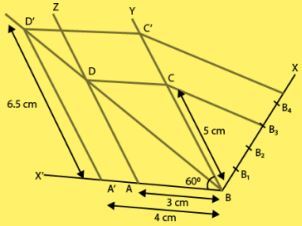3.  Draw two concentric circles of radii 3 cm and 5 cm. Taking a point on outer circle construct the pair of tangents to the other. Measure the length of a tangent and verify it by actual calculation

Solution

We have, two concentric circles of radii 3 cm and 5 cm with centre O.
We draw pair of tangents from point P on outer circle to the other.

1. Draw two concentric circles with centre O and radii 3 cm and 5 cm.
2. Taking any point P on outer circle. Join OP.
3. Bisect OP, let M’ be the mid-point of OP taking M’ as centre and OM’ as radius draw a circle dotted which cuts the inner circle at M and P’.
4. Join PM and PP’. Thus, PM and PP’ are the required tangents.
5. On measuring PM and PP’, we find that PM = PP’ = 4 cm.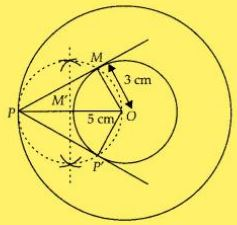Now actual calculation:
In right angle ∆OMP,
∠PMO = 90°
PM2 = OP2 – OM2   [by Pythagoras theorem]

PM2 = (5)2 – (3)2

⇒ 25 – 9 = 16
⇒ PM = 4 cm

Therefore, the length of both tangents is 4 cm.

4. Draw an isosceles triangle ABC in which AB = AC = 6 cm and BC = 5 cm. Construct a triangle PQR similar to triangle ABC in which PQ = 8 cm. Also justify the construction.

Solution

Let ∆PQR and ∆ABC are similar triangles, then its scale factor between the corresponding sides is
PQ/AB = 8/6 = 4/3
Steps of construction :

1. Draw a line segment BC = 5 cm.
2. Construct OQ the perpendicular bisector of line segment BC meeting BC at P’.
3. Taking B and C as centre we draw two arcs of equal radius 6 cm intersecting each other at A.
4. Join BA and CA. So, ∆ABC is the required isosceles triangle.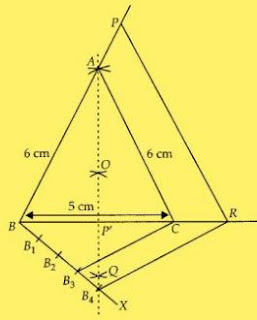5. From B, we draw any ray BX making an acute ∠CBX.
6. Locate four points B1 , B2 , B3 and B4  on BX such that BB1 = B1B2 = B2B3 = B3B4 .
7. Now join B3C and from B4 draw a line B4R ॥ B3C intersecting the extended line segment BC at R.
8. From point R, draw RP ॥ CA meeting BA produced at P.
Then, ∆ PBR is the required triangle.
Explanation,
As, we have,
B4R || B3(by construction)
BC/CR = 3/1
or,
CR/BC = 1/3
Now,
BR/BC = (BC + CR)/BC
= 1 + (CR/BC)
= 1 + (1/3)
= 4/3
And,
RP || CA
So, ∆ABC ～∆PBR
Hence,
PB/AB = RP/CA = BR/BC = 4/3

5. Draw a triangle ABC in which AB = 5 cm, BC = 6 cm and ABC = 60. Construct a triangle similar to ABC with scale factor 5/7 . Justify the construction.

Solution

Steps of construction:

1. Draw a line segment AB = 5 cm.
2. From point B, draw ∠ABY = 60° on which take BC = 6 cm.
3. Join AC, ∆ABC is the required triangle.
4. From A, draw any ray AX downwards making an acute angle ∠BAX
5. Mark 7 points B1 , B2 , B3 , B4 , B5 , B6 and B7  on AX, such that AB1 = B1B2 = B2B3 = B3B4  = B4B5 = B5B6 = B6B7 .
6. Join B7B and from B5 draw B5M ॥ B7B intersecting AB at M.
7. From point M draw MN ॥ BC intersecting AC at N. Then, ∆AMN is the required triangle whose sides are equal to 5/7 of the corresponding sides of the ∆ABC.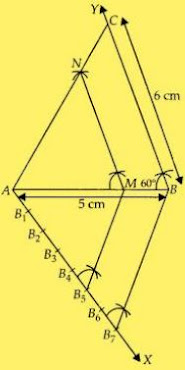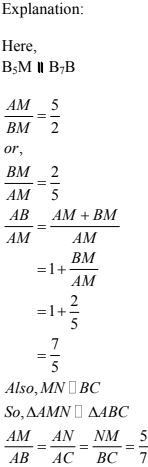6. Draw a circle of radius 4 cm. Construct a pair of tangents to it, the angle between which is 60°. Also justify the construction. Measure the distance between the centre of the circle and the point of intersection of tangents.

Solution

Steps of construction:

1. Take a point O on the plane of the paper and draw a circle of radius OA = 4 cm.
2. Produce OA to B such that OA = AB = 4 cm.
3. Taking A as the centre draw a circle of radius AO = AB = 4 cm.
Suppose it cuts the circle drawn in step 1 at P and Q.
4. Join BP and BQ to get desired tangents.
Explanation:
In ∆OAP, we have
OA = OP = 4 cm (Radius)
Also,
AP = 4 cm
(As, Radius of circle with centre A)
∆OAP is equilateral triangle.
∠PAO = 60°
∠BAP = 120°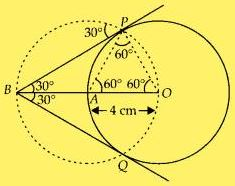Therefore in ∆BAP,
BA = AP
and ∠BAP = 120°
So,
∠ABP = ∠APB = 30°
∠PBQ = 60°

7. Draw a triangle ABC in which AB = 4 cm, BC = 6 cm and AC = 9 cm. Construct a triangle similar to ∆ABC with scale factor 3/2 . Justify the construction. Are the two triangles congruent? Note that all the three angles and two sides of the two triangles are equal.
Solution
Steps of construction:
1. Firstly draw a line segment BC = 6 cm.
2. Taking B and C as centre, draw two arcs of radii 4 cm and 9 cm intersecting each other at A.
3. Join BA and CA. ∆ABC is the required triangle.
4. From B, draw any ray BX downwards making an acute angle ∠CBX
5. Mark three points B1 , B2 , B3 , on BX, such that BB1 = B1B2 = B2B3.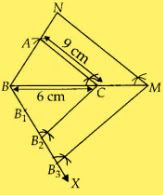6. Join B2C and from B3 draw B3M ॥ B2C intersecting BC at M.
7. From point M, draw MN ॥ CA intersecting the extended line segment BA to N.
Then ∆NBM is the required triangle whose sides are equal to 3/2 of the ΔABC.
Explanation :
B3M ॥ B2C
BC/CM = 2/1
Now,
BM/BC = (BC + CM)/BC
= 1 + (CM/BC)
= 1 + (1/2)
= 3/2
Also,
MN || CA
ΔABC : ΔNBM
So,
NB/AB = NM/AC = BM/BC = 3/2
The two triangles are not congruent because, if two triangles are congruent, then they have
same shape and same size.
Therefore, all the three angles are same but three sides are not same that is one side is
different.